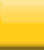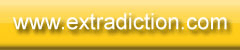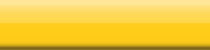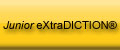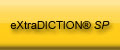Home Junior eXtraDICTION® eXtraDICTION® SP Others Contact Us If you have any enquiries, please write to us at contact@extradiction.com You can also write to us at our snail mail address: Ed-Infinitum (M) Sdn Bhd 25A Lorong Datuk Sulaiman 7 Taman Tun Dr. Ismail 60000 Kuala Lumpur MALAYSIA

 “The basic idea of the game is to guess words or phrases from the definitions given and then to write the correct answers on the game board to get the most points.” To play the "Classic" game, you’ll need: the eXtraDICTION® game board; the eXtraDICTION® playing cards; the Word Insertion Guides (WIG); dry-erase (whiteboard) markers and eraser; paper and pencil (to keep score). click here to learn more about the components of the eXtraDICTION® SP game set You’ll also need: 2 to 4 players. General Game Flow Let’s say there are four players playing the game. Player 1 starts the game: she asks a question;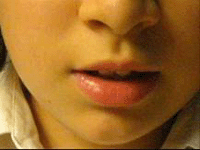the other players try to answer;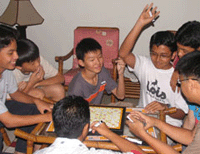the answer is then written on the game board;the score for the answer is calculated. Player 2 now takes her turn to ask a question and the other players try to answer, and so on. A round is completed when each of the players has had a turn to ask a question. The game ends after 5 rounds (or more rounds subject to all players agreeing at the outset of the game). The player with the highest number of points wins. *** You can also view a narrated slide presentation on how to play eXtraDICTION SP by clicking here. Note that some browsers may experience intermittent technical difficulties. The FAQ section below will help you understand the rules of the game better. Asking the Question Q: Who does the questioning? A: In every round, each player has a turn to ask a question. Q: Where do the questions come from? A: The questions (clues) are on the back of the playing cards. The player asking the question picks a card from the pack, chooses one question from that card and reads it aloud. [The answers are on the front of the cards].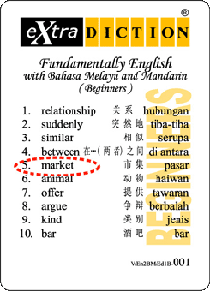Q: How is the question asked? A: If a question (clue) on the back of the card says, “m (6) the place with many small stalls selling different goods”, the player says, “6-letter word beginning with ‘m’; the place with many small stalls selling different goods”. [The answer is ‘market’.]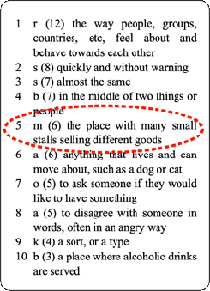Q: Is the player asking the question allowed to see the answer before he chooses the question? A: Yes. Answering Q: Who gets to answer first? A: The player who puts her hand up first answers, but only when the player who asked the question tells her to. If the answer is wrong, the next player to put up her hand gets to answer, and so on. Q: How much time do the players get to answer? A: All the players have a total of 30 seconds between them once the player asking the question has finished reading aloud the clue. However, each player is allowed only 5 seconds to answer from the time the player asking the question tells him to. Q: How many times is a player allowed to answer? A: During each turn, each player is allowed to answer once only. Q: What happens if a player gives more than one answer when it is her turn to answer? A: Only the first answer is allowed. The rest are ignored. Q: What happens if a player answers when it is NOT her turn to answer? A: The answers are ignored and she loses her turn to answer. Q: Can another player use an answer that was ignored? A: Yes. Q: When it is a player’s turn to answer, what happens after she gives the correct answer? A: She writes the answer on the game board and scores some points. Q: What happens if none of the players gives the correct answer? A: The player who asked the question for that turn writes the answer on the board and scores some points. Writing on the Board Q: What are the basic rules for writing the answer on the board? A: Answers must be written in a single horizontal line from left to right or a single vertical line from top to bottom only. Each character (letter or punctuation mark) has to use one square. If the answer is a phrase, blank squares are left for the spaces between the words.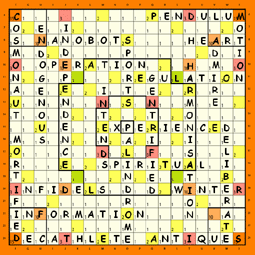Q: Can you write the answers diagonally? A: No. You are also not allowed to: - write horizontally from right to left; - write vertically from bottom to top; - change direction midway through a word or phrase; - create non-existent words or phrases; - modify an existing word or phrase to become an non-existent word or phrase in the language the game is being played.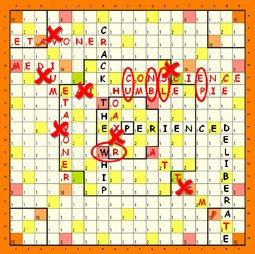Q: Can the first answer be written anywhere on the board? A: No. One character of the first answer written must make use the square that is at the centre of the board. Q: What about the answers after that? A: They may:- 1. Cross existing answers; 2. Touch existing answers; or 3. Float, that is, not cross or touch any existing answer. Note: When a new answer touches an existing answer any new word formed must be an actual word that can be found in a dictionary. Q: How much time does a player have to finish writing an answer on the board? A: 30 seconds. Q: What happens if, 1) a player does not finish writing the answer in the time allowed, or 2) the spelling is wrong, or 3) any new word formed is not an actual word? A: Before the next turn has started, the other players can have the answer removed from the board and any points scored for that answer is not counted. Otherwise, the answer and the score remain. Scoring Q: How are points scored? A: Except for a “floating” answer, the normal method of calculating the score for an answer is:- the sum of the values of the squares occupied by an answer, multiplied by the number of zones occupied by that answer.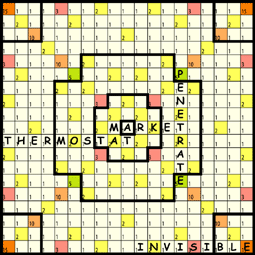The word "MARKET" occupies a total of 6 squares. In this example it occupies 5 white squares with square values of 1 each and 1 yellow square with a square value of 2. So the sum of the square values occupied by the word "MARKET" is 1+1+1+2+1+1=7.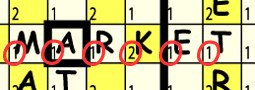The word "market also occupies 3 zones. Therefore the score for the word "MARKET" in this example is 7 x 3 = 21. The next word "PENETRATE" crosses the word "MARKET" at the letter "T". The score is 19 x 2 = 38. The third word, “THERMOSTAT”, touches “MARKET” at the letters “M” and “A” and forms two new words, “MA” and “AT”. The score for the turn is: “THERMOSTAT” : 12 x 3 = 36 “MA” : 3 x 1 = 3 “AT” : 2 x 2 = 4 Total = 43 Q: How do you score a “floating” answer? A: The score is the sum of the values of the squares occupied by the answer only. You do not multiply it by the number of zones occupied. In the example above, the fourth word, “INVISIBLE” is floating. The score is 26. Q: Is the first answer written on the board considered a “floating” answer? A: No. (Therefore it is scored using the normal method.) Q: What do you mean by ”the sum of the values of the squares occupied by an answer”? A: Every square on the game board has a value printed in it. The squares are colour-coded for easy recognition.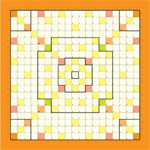Q: Where are the zones on the board? A: The bold lines on the board denote the boundary of the zones. Zone 1 is in the centre of the board and has one square only. Surrounding it is Zone 2 which has 20 squares, and so on for Zone3, Zone 4 and the Corner Zones. There are a total of 8 zones on the board. Q: What happens if an answer occupies the same zone twice? A: For the purpose of scoring, that zone is counted once only. Q: When an answer touches an existing answer and new words are created, do you get points for the newly created words as well? A: Yes. Others Q: The example above is for 4 players. What happens when only 2 or 3 players play? A: When there are 2 players, the game lasts 10 rounds. When there are 3 players, the game lasts 7 rounds. Q: Can more than 4 people play the a game? A: Yes, although it might affect your enjoyment of the game a little bit. You may have to reduce the number of rounds played because you might run out of squares to write the answers. Q: How do you play SPEED eXtraDICTION®? A: The rules are the same as the Classic game except that there are no questions or answers. Players simply take turns to write their own words or phrases on the board. It is essential that you have a good dictionary handy. Q: Are there other ways of playing with the eXtraDICTION® SP game set? A: Yes. Click here to learn more. back to top